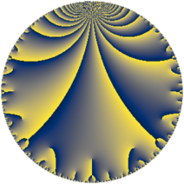# Properties

 Label 165.6.cLevel $165$ Weight $6$ Character orbit 165.c Rep. character $\chi_{165}(34,\cdot)$ Character field $\Q$ Dimension $52$ Newform subspaces $2$ Sturm bound $144$ Trace bound $4$

# Related objects

## Defining parameters

 Level: $$N$$ $$=$$ $$165 = 3 \cdot 5 \cdot 11$$ Weight: $$k$$ $$=$$ $$6$$ Character orbit: $$[\chi]$$ $$=$$ 165.c (of order $$2$$ and degree $$1$$) Character conductor: $$\operatorname{cond}(\chi)$$ $$=$$ $$5$$ Character field: $$\Q$$ Newform subspaces: $$2$$ Sturm bound: $$144$$ Trace bound: $$4$$

## Dimensions

The following table gives the dimensions of various subspaces of $$M_{6}(165, [\chi])$$.

Total New Old
Modular forms 124 52 72
Cusp forms 116 52 64
Eisenstein series 8 0 8

## Trace form

 $$52 q - 876 q^{4} - 196 q^{5} + 180 q^{6} - 4212 q^{9} + O(q^{10})$$ $$52 q - 876 q^{4} - 196 q^{5} + 180 q^{6} - 4212 q^{9} - 1708 q^{10} + 6736 q^{14} + 15284 q^{16} + 80 q^{19} + 6524 q^{20} + 360 q^{21} - 540 q^{24} + 10996 q^{25} + 9864 q^{26} - 14208 q^{29} + 2736 q^{30} + 38144 q^{31} - 8432 q^{34} + 5760 q^{35} + 70956 q^{36} - 11160 q^{39} + 5212 q^{40} + 40128 q^{41} + 4840 q^{44} + 15876 q^{45} - 48800 q^{46} - 192788 q^{49} - 52712 q^{50} - 10440 q^{51} - 14580 q^{54} - 273600 q^{56} + 149568 q^{59} + 113112 q^{60} - 93208 q^{61} - 585068 q^{64} - 73960 q^{65} + 34848 q^{66} - 98496 q^{69} + 169704 q^{70} + 47760 q^{71} + 452704 q^{74} + 38880 q^{75} - 262416 q^{76} + 36608 q^{79} + 171988 q^{80} + 341172 q^{81} - 54432 q^{84} - 87400 q^{85} + 103360 q^{86} + 628456 q^{89} + 138348 q^{90} + 300672 q^{91} + 587248 q^{94} - 243408 q^{95} - 476100 q^{96} + O(q^{100})$$

## Decomposition of $$S_{6}^{\mathrm{new}}(165, [\chi])$$ into newform subspaces

Label Dim $A$ Field CM Traces $q$-expansion
$a_{2}$ $a_{3}$ $a_{5}$ $a_{7}$
165.6.c.a $26$ $26.463$ None $$0$$ $$0$$ $$-98$$ $$0$$
165.6.c.b $26$ $26.463$ None $$0$$ $$0$$ $$-98$$ $$0$$

## Decomposition of $$S_{6}^{\mathrm{old}}(165, [\chi])$$ into lower level spaces

$$S_{6}^{\mathrm{old}}(165, [\chi]) \cong$$ $$S_{6}^{\mathrm{new}}(5, [\chi])$$$$^{\oplus 4}$$$$\oplus$$$$S_{6}^{\mathrm{new}}(15, [\chi])$$$$^{\oplus 2}$$$$\oplus$$$$S_{6}^{\mathrm{new}}(55, [\chi])$$$$^{\oplus 2}$$